# Formula Errors

This chapter teaches you how to deal with some common formula errors in Excel. Let's start simple.

### #####

When your cell contains this error code, the column isn't wide enough to display the value.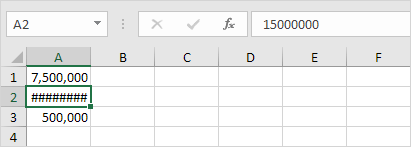1. Click on the right border of the column A header and increase the column width.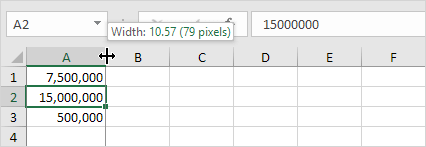Tip: double click the right border of the column A header to automatically fit the widest entry in column A.

### #NAME?

The #NAME? error occurs when Excel does not recognize text in a formula.1. Simply correct SU to SUM.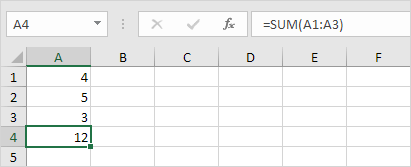### #VALUE!

Excel displays the #VALUE! error when a formula has the wrong type of argument.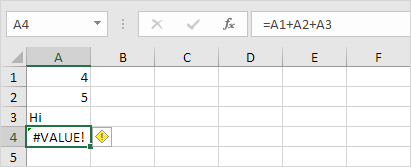1a. Change the value of cell A3 to a number.
1b. Use a function to ignore cells that contain text.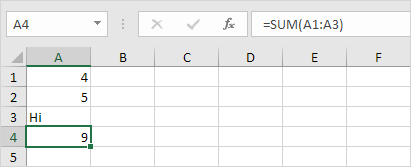### #DIV/0!

Excel displays the #DIV/0! error when a formula tries to divide a number by 0 or an empty cell.1a. Change the value of cell A2 to a value that is not equal to 0.
1b. Prevent the error from being displayed by using the logical function IF.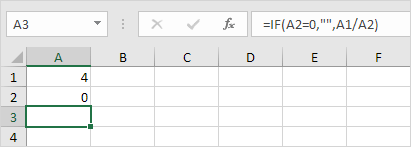Explanation: if cell A2 equals 0, an empty string ("") is displayed. If not, the result of the formula A1/A2 is displayed.

### #REF!

Excel displays the #REF! error when a formula refers to a cell that is not valid.

1. Cell C1 references cell A1 and cell B1.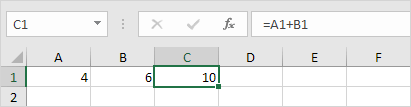2. Delete column B. To achieve this, right click the column B header and click Delete.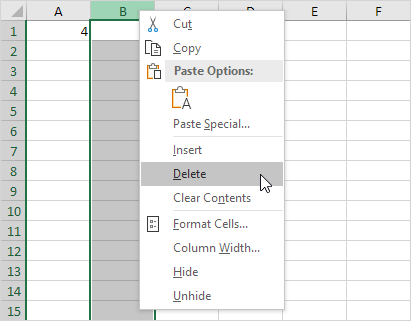3. Select cell B1. The reference to cell B1 is not valid anymore.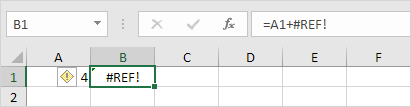4. To fix this error, you can either delete +#REF! in the formula of cell B1 or you can undo your action by pressing CTRL + z

### #N/A

The #N/A error appears when the VLOOKUP function (or XLOOKUP, MATCH, etc.) can't find a match.

1. In the example below, ID 28 cannot be found.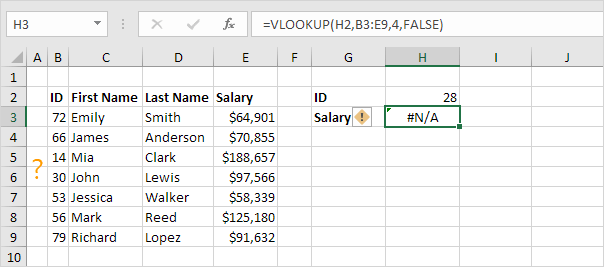2. Use the IFNA function to replace the #N/A error with a friendly message.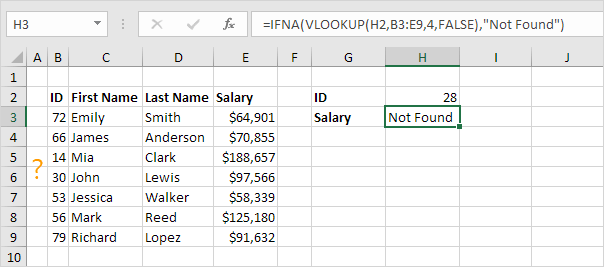### #NUM!

Excel shows the #NUM! error when a formula contains invalid numeric values.

1. For example, the SQRT function below cannot calculate the square root of a negative number.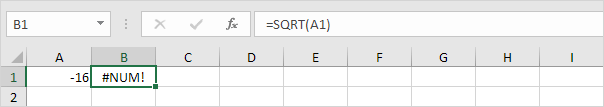2. Change the number in cell A1 to a positive number.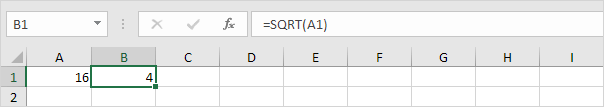### #NULL!

The intersect operator (single space) returns the intersection of two ranges. When two ranges don't intersect, Excel displays the #NULL! error.

1. The formula below returns #NULL! because the two ranges don't intersect.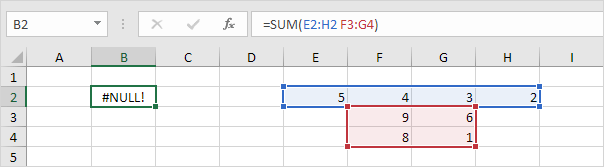2. The formula below doesn't return the #NULL error.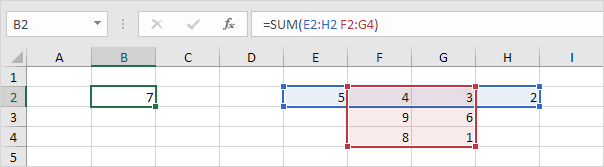Note: =SUM(F2:G2) produces the exact same result!

### #SPILL!

If something is blocking a spill range, Excel displays the #SPILL! error.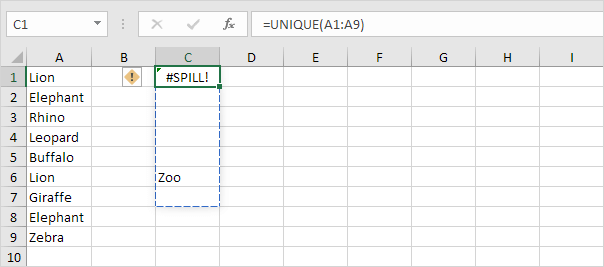1. Simply empty cell C6 to fix the #SPILL error.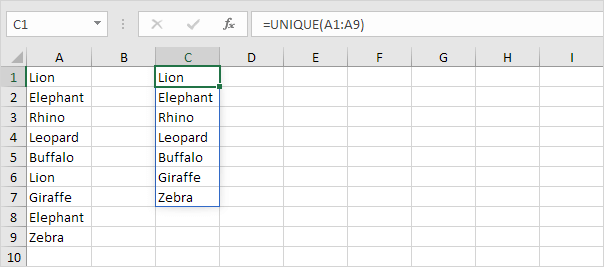Note: this dynamic array function, entered into cell C1, fills multiple cells. Wow! This behavior in Excel 365/2021 is called spilling.

Go to Next Chapter: Array Formulas